# Arduino Touch Screen Calculator using TFT LCD

Published  August 24, 2017   16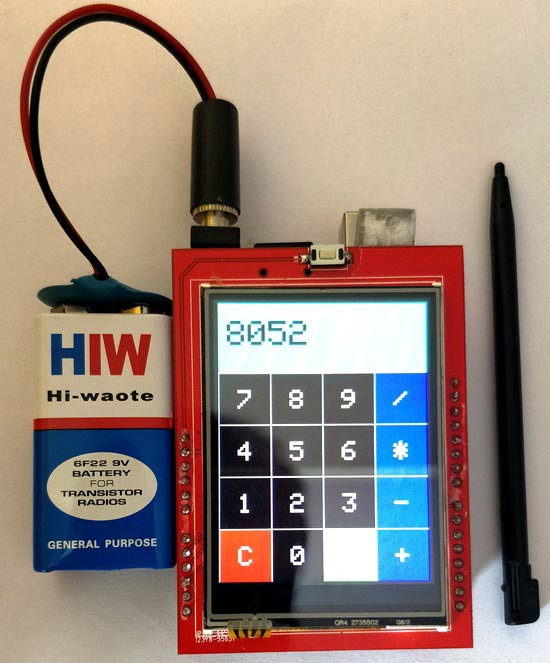Arduino has always helped to build projects easily and make them look more attractive.  Programming an LCD screen with touch screen option might sound as a complicated task, but the Arduino libraries and shields had made it really easy. In this project we will use a 2.4” Arduino TFT LCD screen to build our own Arduino Touch Screen calculator that could perform all basic calculations like Addition, Subtraction, Division and Multiplication.

### Materials Required:

1. Arduino Uno
2. 2.4” TFT LCD display Shield
3. 9V Battery.

### Getting to know the TFT LCD Screen Module:

Before we actually dive into the project it is important to know, how this 2.4” TFT LCD Module works and what are the types present in it. Let us take a look at the pinouts of this 2.4” TFT LCD screen module.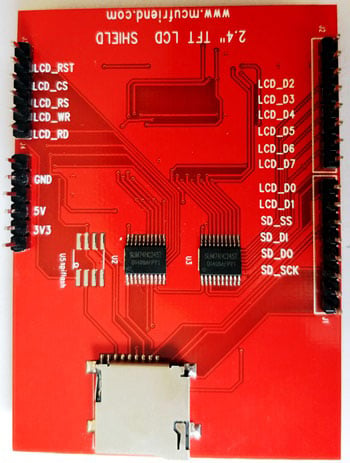As you can see there are 28 pins which will perfectly fit into any Arduino Uno / Arduino Mega Board. A small classification of these pins is given in the table below.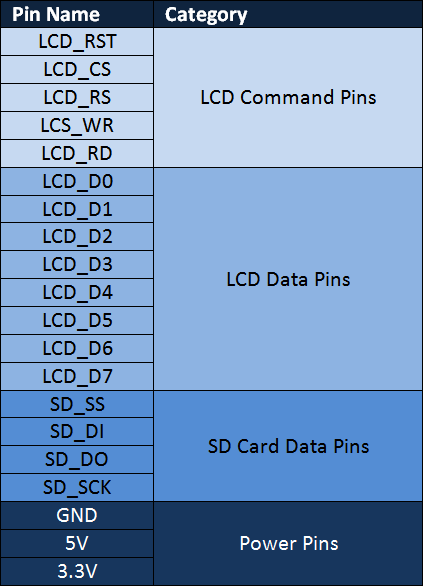As you can see the pins can be classified in to four main classifications such as LCD Command Pins, LCD Data Pins, SD Card Pins and Power Pins, We need not know much about the detailed working of these pins since they will be take care by our Arduino Library.

You can also find an SD card slot at the bottom of the module shown above, which can be used to load an SD card with bmp image files, and these images can be displayed in our TFT LCD screen using the Arduino Program.

Another important thing to note is your Interface IC. There are many types of TFT modules available in the market starting from the original Adafruit TFT LCD module to cheap Chinese clones. A program which works perfectly for your Adafruit shield might not work the same for Chinese breakout boards. So, it is very important to know which types of LCD display your are holding in hand. This detail has to be obtained from the vendor. If you are having a cheap clone like mine then it is most probably using the ili9341 driver IC. You can follow this TFT LCD interfacing with Arduino tutorial to try out some basic example programs and get comfortable with the LCD screen. Also check out our other TFT LCD projects with Arduino here:

### Calibrating the TFT LCD Screen for Touch Screen:

If you planning to use the touch screen function of your TFT LCD module, then you have to calibrate it to make it work properly.  A LCD screen without calibration might work unlikely, for instance you might touch at one place and the TFT might respond for a touch at some other place. These calibrations results will not be similar for all boards and hence you are left on your own to do this.

The best way to calibrate is to use the calibration example program (comes with library) or use the serial monitor to detect your error.  However for this project since the size of buttons is large calibration should not be a big problem and I will also explain how you can calibrate your screen under the programming section below.

### TFT LCD Connections with Arduino:

The 2.4” TFT LCD screen is a perfect Arduino Shield. You can directly push the LCD screen on top of the Arduino Uno and it will perfectly match with the pins and slid in through. However, as matters of safety cover the Programming terminal of your Arduino UNO with a small insulation tape, just in case if the terminal comes in contact with your TFT LCD screen. The LCD assembled on UNO will look something like this below.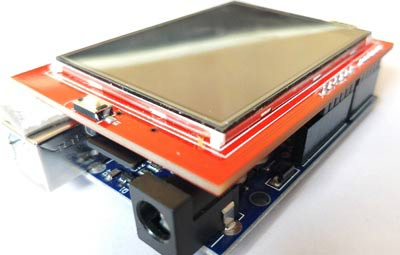### Programming your Arduino for TFT LCD:

We are using the SPFD5408 Library to get this arduino calculator code working. This is a modified library of Adafruit and can work seamlessly with our LCD TFT Module. You can check the complete program at the end of this Article.

Note: It is very important for you to install this library in your Arduino IDE or this program to compile without any error.

Now, open Arduino IDE and select Sketch -> Include Librarey -> Add .ZIP library. A browser window will open navigate to the ZIP file and click “OK”. You should notice “Library added to your Libraries” on the bottom-left corner of Arduino, if successful. A detailed guide to do the same is given in the Interfacing Tutorial.

Now, you can use the code below in your Arduino IDE and upload it to your Arduino UNO for the Touch Screen Calculator to work. Further down, I have explained the code into small segments.

We need three libraries for this program to work; all these three libraries were given in the ZIP file you downloaded from the above provided link. I have simply included them in the code as shown below.

```#include <SPFD5408_Adafruit_GFX.h>    // Core graphics library
#include <SPFD5408_TouchScreen.h>```

As said earlier we need to calibrate the LCD screen to make it work as expected, but don’t worry the values given here are almost universal. The variables TS_MINX, TS_MINY, TS_MAXX, and TS_MAXY decide the calibration of the Screen. You can toy around them if you feel the calibration is not satisfactory.

```#define TS_MINX 125
#define TS_MINY 85
#define TS_MAXX 965
#define TS_MAXY 905```

As we know the TFT LCD screen can display a lot of colours, all these colours have to be entered in hex value. To make it more human readable we assign these values to a variable as shown below.

Note: The values are true only if the screen is rotate by 2. This is for programming convenience.

```#define WHITE   0x0000 //Black->White
#define YELLOW    0x001F //Blue->Yellow
#define CYAN     0xF800 //Red->Cyan
#define PINK   0x07E0 //Green-> Pink
#define RED    0x07FF //Cyan -> Red
#define GREEN 0xF81F //Pink -> Green
#define BLUE  0xFFE0 //Yellow->Blue
#define BLACK   0xFFFF //White-> Black```

Okay now, we can get into the programming part. There are three sections involved in this program. One is creating a UI of a calculator with buttons and display. Then, detecting the buttons based on the users touch and finally calculating the results and display them. Let us get through them one by one.

1. Creating an UI of Calculator:

This is where you can use a lot of your creativity to design the User Interface of calculator. I have simply made a basic layout of a calculator with 16 Buttons and one display unit. You have to construct the design just like you will draw something on MS paint. The libraries added will allow you to draw Lines, Rectangle, Circles, Chars, Strings and lot more of any preferred colour. You can understand the available functions from this article.

I have used the line and box drawing abilities to design an UI which looks very similar to the 90’s calculator.  Each box has a width and height of 60 pixels.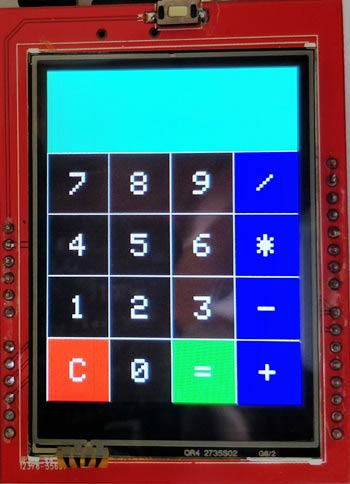```//Draw the Result Box
tft.fillRect(0, 0, 240, 80, CYAN);

//Draw First Column
tft.fillRect  (0,260,60,60,RED);
tft.fillRect  (0,200,60,60,BLACK);
tft.fillRect  (0,140,60,60,BLACK);
tft.fillRect  (0,80,60,60,BLACK);

//Draw Third Column
tft.fillRect  (120,260,60,60,GREEN);
tft.fillRect  (120,200,60,60,BLACK);
tft.fillRect  (120,140,60,60,BLACK);
tft.fillRect  (120,80,60,60,BLACK);

//Draw Secound & Fourth Column
for (int b=260; b>=80; b-=60)
{ tft.fillRect  (180,b,60,60,BLUE);
tft.fillRect  (60,b,60,60,BLACK);}

//Draw Horizontal Lines
for (int h=80; h<=320; h+=60)
tft.drawFastHLine(0, h, 240, WHITE);

//Draw Vertical Lines
for (int v=0; v<=240; v+=60)
tft.drawFastVLine(v, 80, 240, WHITE);

for (int j=0;j<4;j++) {
for (int i=0;i<4;i++) {
tft.setCursor(22 + (60*i), 100 + (60*j));
tft.setTextSize(3);
tft.setTextColor(WHITE);
tft.println(symbol[j][i]);```

2. Detecting the buttons:

Another challenging task is detecting the user touch. Every time the user touches somewhere we will able to how where the X and Y position of the pixel he touched. This value can be displayed on the serial monitor using the println as shown below.

```TSPoint p = waitTouch();
X = p.y; Y = p.x;
Serial.print(X); Serial.print(','); Serial.println(Y);// + " " + Y);```

Since we have designed the box with width and height of 60 pixel each and have four Rows and for columns starting from (0,0). The position of each box can be predicted as shown in below picture.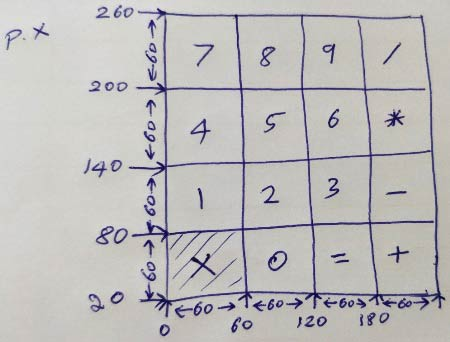But in practical case, this will not be the result. There will be a big difference between the expected and actual value, due to the calibration problem.

So, to predict the exact position of the box, you have to click on the line and check its corresponding position on the serial monitor. This might not be most professional way of doing it, but still it works perfectly. I measured the position of all the lines and obtained the below values.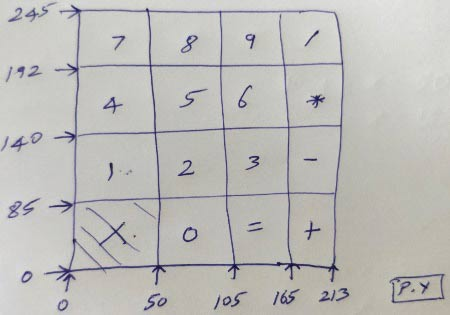Now, since we know the position of all the boxes. When a user touches anywhere we can predict where he has touched by comparing his (X,Y) values with the value for each box as shown below.

```if (X<105 && X>50) //Detecting Buttons on Column 2
{
if (Y>0 && Y<85)
{Serial.println ("Button 0"); //Button 0 is Pressed
if (Number==0)
Number=0;
else
Number = (Number*10) + 0; //Pressed twice
}

if (Y>85 && Y<140)
{Serial.println ("Button 2");
if (Number==0)
Number=2;
else
Number = (Number*10) + 2; //Pressed twice
}```

3. Displaying Numbers and Calculating the Result:

The final step is to calculate the result and display them on TFT LCD Screen. This arduino calculator can perform operation with 2 numbers only. These two numbers are named as variables “Num1” and “Num2”. The variable “Number” gives and takes value from Num1 and Num2 and also bears the result.

When a use presses a button, one digit is added to number. When another button is pressed, the previous one digit is multiplied with 10 and the new number is added with it. For example, if we press 8 and then press 5 and then press 7. Then first the variable will hold 8 then (8*10)+5=85 then (85*10)+7 = 857. So finally the variable will have the value 857 with it.

```if (Y>192 && Y<245)
{Serial.println ("Button 8");
if (Number==0)
Number=8;
else
Number = (Number*10) + 8; //Pressed again
}  ```

When we perform any operation like addition, when the users presses the addition button the value from Number will be transferred to Num1 and then Number will be made zero so that it gets ready to take the input for second number.

When Equal is pressed the value in Number will be sent to Num2 and then the respective calculation (in this case addition) will be made and the result will be again stored in the variable “Number”.

Finally this value will be displayed in the LCD screen.

### Working:

The working of this Arduino Touch Screen Calculator is simple. You have to upload the below given code on your Arduino and fire it up. You get the calculator displayed on your LCD screen.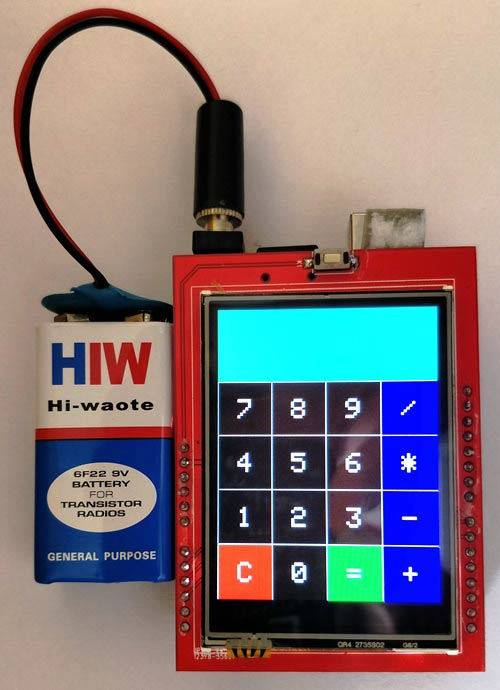Now, you can enter any number and perform your calculations. It is limited to only two operand and only operator for now. But, you can tweak the code to make it have lots of option.

You have to press the “C” to clear the value on screen each time after performing a calculation. Hope you understood the project and enjoyed building something similar. If you have any doubts feel free to post them on forums or on the comment section below. See you next time with another interesting project until then happy computing!!

Also check the Demonstration Video Below.

Code

/*______Import Libraries_______*/
#include <SPFD5408_Adafruit_GFX.h>    // Core graphics library
#include <SPFD5408_TouchScreen.h>
/*______End of Libraries_______*/

/*______Define LCD pins (I have asigned the default values)_______*/
#define YP A1  // must be an analog pin, use "An" notation!
#define XM A2  // must be an analog pin, use "An" notation!
#define YM 7   // can be a digital pin
#define XP 6   // can be a digital pin
#define LCD_CS A3
#define LCD_CD A2
#define LCD_WR A1
#define LCD_RD A0
#define LCD_RESET A4
/*_______End of defanitions______*/

/*______Assign names to colors and pressure_______*/
#define WHITE   0x0000 //Black->White
#define YELLOW    0x001F //Blue->Yellow
#define CYAN     0xF800 //Red->Cyan
#define PINK   0x07E0 //Green-> Pink
#define RED    0x07FF //Cyan -> Red
#define GREEN 0xF81F //Pink -> Green
#define BLUE  0xFFE0 //Yellow->Blue
#define BLACK   0xFFFF //White-> Black
#define MINPRESSURE 10
#define MAXPRESSURE 1000
/*_______Assigned______*/

/*____Calibrate TFT LCD_____*/
#define TS_MINX 125
#define TS_MINY 85
#define TS_MAXX 965
#define TS_MAXY 905
/*______End of Calibration______*/

TouchScreen ts = TouchScreen(XP, YP, XM, YM, 300); //300 is the sensitivity
Adafruit_TFTLCD tft(LCD_CS, LCD_CD, LCD_WR, LCD_RD, LCD_RESET); //Start communication with LCD

String symbol = {
{ "7", "8", "9", "/" },
{ "4", "5", "6", "*" },
{ "1", "2", "3", "-" },
{ "C", "0", "=", "+" }
};
int X,Y;
long Num1,Num2,Number;
char action;
boolean result = false;

void setup() {
Serial.begin(9600); //Use serial monitor for debugging
tft.reset(); //Always reset at start
tft.begin(0x9341); // My LCD uses LIL9341 Interface driver IC
tft.setRotation(2); // I just roated so that the power jack faces up - optional
tft.fillScreen(WHITE);

IntroScreen();

draw_BoxNButtons();
}

void loop() {
TSPoint p = waitTouch();
X = p.y; Y = p.x;
//  Serial.print(X); Serial.print(','); Serial.println(Y);// + " " + Y);

DetectButtons();

if (result==true)
CalculateResult();

DisplayResult();

delay(300);
}

TSPoint waitTouch() {
TSPoint p;
do {
p = ts.getPoint();
pinMode(XM, OUTPUT);
pinMode(YP, OUTPUT);
} while((p.z < MINPRESSURE )|| (p.z > MAXPRESSURE));
p.x = map(p.x, TS_MINX, TS_MAXX, 0, 320);
p.y = map(p.y, TS_MINY, TS_MAXY, 0, 240);;
return p;
}

void DetectButtons()
{

if (X<50 && X>0) //Detecting Buttons on Column 1
{
if (Y>0 && Y<85) //If cancel Button is pressed
{Serial.println ("Button Cancel"); Number=Num1=Num2=0; result=false;}

if (Y>85 && Y<140) //If Button 1 is pressed
{Serial.println ("Button 1");
if (Number==0)
Number=1;
else
Number = (Number*10) + 1; //Pressed twice
}

if (Y>140 && Y<192) //If Button 4 is pressed
{Serial.println ("Button 4");
if (Number==0)
Number=4;
else
Number = (Number*10) + 4; //Pressed twice
}

if (Y>192 && Y<245) //If Button 7 is pressed
{Serial.println ("Button 7");
if (Number==0)
Number=7;
else
Number = (Number*10) + 7; //Pressed twice
}
}

if (X<105 && X>50) //Detecting Buttons on Column 2
{
if (Y>0 && Y<85)
{Serial.println ("Button 0"); //Button 0 is Pressed
if (Number==0)
Number=0;
else
Number = (Number*10) + 0; //Pressed twice
}

if (Y>85 && Y<140)
{Serial.println ("Button 2");
if (Number==0)
Number=2;
else
Number = (Number*10) + 2; //Pressed twice
}

if (Y>140 && Y<192)
{Serial.println ("Button 5");
if (Number==0)
Number=5;
else
Number = (Number*10) + 5; //Pressed twic
}

if (Y>192 && Y<245)
{Serial.println ("Button 8");
if (Number==0)
Number=8;
else
Number = (Number*10) + 8; //Pressed twic
}
}

if (X<165 && X>105) //Detecting Buttons on Column 3
{
if (Y>0 && Y<85)
{Serial.println ("Button Equal");
Num2=Number;
result = true;
}

if (Y>85 && Y<140)
{Serial.println ("Button 3");
if (Number==0)
Number=3;
else
Number = (Number*10) + 3; //Pressed twice
}

if (Y>140 && Y<192)
{Serial.println ("Button 6");
if (Number==0)
Number=6;
else
Number = (Number*10) + 6; //Pressed twice
}

if (Y>192 && Y<245)
{Serial.println ("Button 9");
if (Number==0)
Number=9;
else
Number = (Number*10) + 9; //Pressed twice
}
}

if (X<213 && X>165) //Detecting Buttons on Column 3
{
Num1 = Number;
Number =0;
tft.setCursor(200, 20);
tft.setTextColor(RED);
if (Y>0 && Y<85)
{Serial.println ("Addition"); action = 1; tft.println('+');}
if (Y>85 && Y<140)
{Serial.println ("Subtraction"); action = 2; tft.println('-');}
if (Y>140 && Y<192)
{Serial.println ("Multiplication"); action = 3; tft.println('*');}
if (Y>192 && Y<245)
{Serial.println ("Devesion"); action = 4; tft.println('/');}

delay(300);
}
}

void CalculateResult()
{
if (action==1)
Number = Num1+Num2;

if (action==2)
Number = Num1-Num2;

if (action==3)
Number = Num1*Num2;

if (action==4)
Number = Num1/Num2;
}

void DisplayResult()
{
tft.fillRect(0, 0, 240, 80, CYAN);  //clear result box
tft.setCursor(10, 20);
tft.setTextSize(4);
tft.setTextColor(BLACK);
tft.println(Number); //update new value
}

void IntroScreen()
{
tft.setCursor (55, 120);
tft.setTextSize (3);
tft.setTextColor(RED);
tft.println("ARDUINO");
tft.setCursor (30, 160);
tft.println("CALCULATOR");
tft.setCursor (30, 220);
tft.setTextSize (2);
tft.setTextColor(BLUE);
tft.println("-Circut Digest");
delay(1800);
}

void draw_BoxNButtons()
{
//Draw the Result Box
tft.fillRect(0, 0, 240, 80, CYAN);

//Draw First Column
tft.fillRect  (0,260,60,60,RED);
tft.fillRect  (0,200,60,60,BLACK);
tft.fillRect  (0,140,60,60,BLACK);
tft.fillRect  (0,80,60,60,BLACK);

//Draw Third Column
tft.fillRect  (120,260,60,60,GREEN);
tft.fillRect  (120,200,60,60,BLACK);
tft.fillRect  (120,140,60,60,BLACK);
tft.fillRect  (120,80,60,60,BLACK);

//Draw Secound & Fourth Column
for (int b=260; b>=80; b-=60)
{ tft.fillRect  (180,b,60,60,BLUE);
tft.fillRect  (60,b,60,60,BLACK);}

//Draw Horizontal Lines
for (int h=80; h<=320; h+=60)
tft.drawFastHLine(0, h, 240, WHITE);

//Draw Vertical Lines
for (int v=0; v<=240; v+=60)
tft.drawFastVLine(v, 80, 240, WHITE);

for (int j=0;j<4;j++) {
for (int i=0;i<4;i++) {
tft.setCursor(22 + (60*i), 100 + (60*j));
tft.setTextSize(3);
tft.setTextColor(WHITE);
tft.println(symbol[j][i]);
}
}
}

Video

Tags

Submitted by Robert Fortier on Fri, 11/17/2017 - 05:30

### Arduino Touch Screen Calculator using TFT LCD

Arduino Touch Screen Calculator using TFT LCD

Submitted by Korben on Sat, 04/21/2018 - 09:32

### This doesn't work on my

This doesn't work on my mcufriend_kbv tft lcd shield. The libraries you provided didn't work. Only the mcufriend library is working

Submitted by Arsalan on Sun, 04/22/2018 - 20:29

### Not working on my spfd 5408

i have uno + SPfd 5408 but white display

Submitted by chinmayee on Sat, 05/12/2018 - 11:26

### the touch is not responding

the touch is not responding what do I do

Submitted by Rahul on Tue, 07/17/2018 - 19:18

### not working

sir, i have done all the things as said above but still the display is showing white only.Please do something.
Thank You

Submitted by narasimharao on Wed, 08/29/2018 - 20:39

### my tuch screen is not responding what i do thanks in advance

my touch screen is not responding what i do thanks in advance

### I need help

I followed the tutorial but after starting the sketch the touch is not working.
After changing the pins:
YP -> A3
XM -> A2
YM -> 9
XP -> 8
I got touch functionality. But it does not work in the right way. When I touch a button field I do not get the right number ot function. The coordinates are not working.

I use a ILI9341 touch TFT with 240 x 320 pixel. Maybe the calibration of my tft is not the same of the tutorial.

What means:
/*____Calibrate TFT LCD_____*/
#define TS_MINX 125
#define TS_MINY 85
#define TS_MAXX 965
#define TS_MAXY 905

Why is:   X = p.y; Y = p.x;

Who can help me???

### several mistakes in your code

Now I fixed my problems. There are several mistakes in your code. I can´t believe that your code worked. If you like, I send you my actual sketch.

Next problem are "decimal numbers".  In the 90s my calculator was able to calculate for example
2.5 * 3.5       or     10 /  4    or    3.5 + 1.7    or     7.3 - 6.9

Your calculator doesn´t know decimal numbers.   ???????

### Hi, I followed your code but

Hi, I followed your code but why whenever I pressed the first button once, the screen will display 2 similar numbers. For example, I pressed on the number '6', and the screen will display '66'. The number I pressed afterwards is working fine just not sure why the first button pressed surely will get twice of it. Hope you know a way to solve it.

### Hello everyone! I followed

Hello everyone! I followed the code and can upload the code on Arduino Mega 2560 only. On Arduino UNO - just white display. There is also no succes on touchscreeen even on Arduino Mega. Maybe someone found a way of uploading the code into Arduino UNO with touchscreen. Thank you in advance for your reply.

### What an interesting sharing,I

What an interesting sharing,I used  TFT LCD by stone,it has a better effect with your code,may be you can have a try

### (No subject)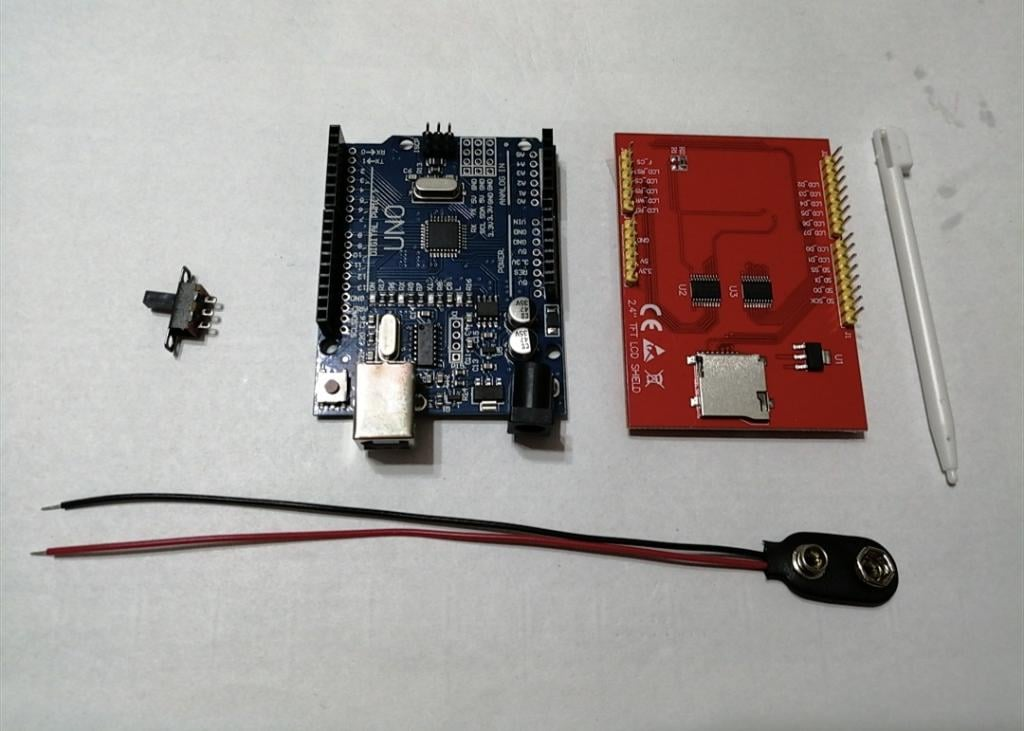### I have modified the code and…

I have modified the code and made it successfully, my video: //youtu.be/UVYKT4yXRc8